# Using IF, AND, OR Functions

In this article, we will delve a little deeper into how to Use IF, AND, OR Functions in Microsoft Excel.

For instructor-led Microsoft Excel training in Los Angeles call us on 888.815.0604..

Logic operations play a big part in Excel’s functionality, especially the IF function. You can use this function to calculate different values depending on the evaluation of a condition. The structure of an IF function is as follows: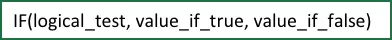IF functions are called conditional functions because the return value will depend on whether or not a specific condition was satisfied. Consider the following function: IF(A1=10,5,1). If the value in A1 equals 10, then 5 is returned. Otherwise, 1 is returned.

For example, in the sample worksheet that you currently have open, fill in cell B1 with this function: IF(A1=10,5,1).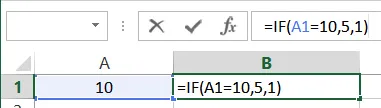With the formula now complete, press Enter to complete the calculation. The value returned will be 5 because the value in A1 is equal to 10: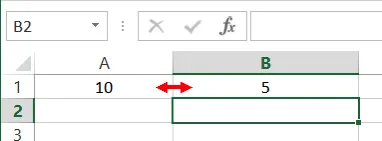Change the value in cell A1 to 9.9. You will see that the IF function will return a value of 1 rather than 5: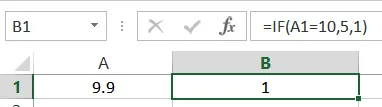Excel’s AND function can work with the IF function to find two (or more) conditions that have to be met, rather than just one. The AND function is structured like this: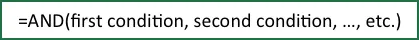Back to the IF statement that you just created, let’s say that you need to display “5” in cell B1 when the value in A1 is greater than or equal to 5, but less than or equal to 9. This means that the AND statement would be nested inside the IF statement, and would look like this: =IF(AND(A1>=5,A1<=9),5,1). Type this new formula into B1: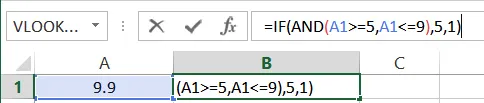Press Enter to apply the new function. The result will immediately be calculated. In this example, because the number in A1 is greater than 9, 1 will be displayed in B1: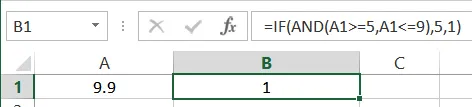Change the number in A1 to 6. The number 5 will be displayed in B1 because it is greater than or equal to 5 AND less than or equal to 9: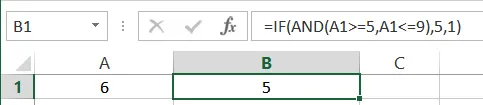Another function you can use with the IF function is OR. This function works similar to the AND statement, but it will be triggered when it finds one condition OR the other, rather than both. The OR function is structured like this: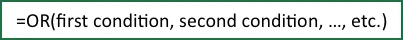Returning to the IF statement that you’ve been working on, let’s say that you need to display 5 in cell B1 when the value in A1 is equal to 1 OR 3. This means that the OR statement would be nested inside the IF statement, and would look like this: =IF(OR(A1=1,A1=3),5,1).

Replace the formula in B1 with this formula: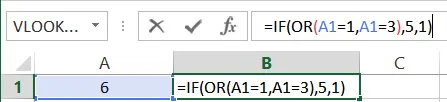Press Enter to apply the new function. The result will be immediately calculated. In this example, because the number in A1 is neither equal to 1 or 3, the number 1 will be displayed in B1: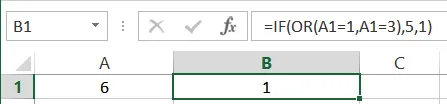Change the value in cell A1 to 3. You will see that the value in B1 will change to 5 because one of the two OR conditions you set in the formula have been met: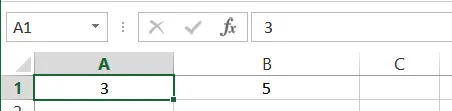#### Microsoft Excel available in LA

Join our 1-day Excel workshop. We run beginner, intermediate and advanced classes every month. Check our past students Excel class reviews.